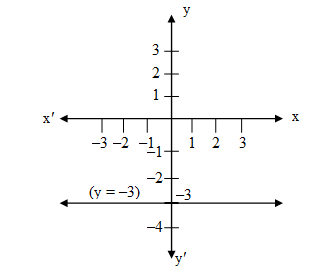Filter By

## All Questions

#### Find the roots of the following quadratic equations by the factorisation method

(ii)

Solution

Hence are the roots of the equation.

(i)

Solution

Hence are the roots of the equation.

(iii)

Solution

Hence are root of the equation

(iv)

Solution

Hence are root of the equation

(v)

solution

Hence are the roots of the equation

#### Find the roots of the quadratic equations by using the quadratic formula in each of the following:

(i)

Compare with  where

Here    a=2, b=-3, c=-5

are the roots of the equation

(ii)

Compare with  where

Here    a=5, b=13, c=8

are the roots of the equation

(iii)

Compare with  where

Here    a=-3, b=5, c=12

(iv) 5,2

Compare with  where

Here    a=-1, b=7, c=-10

x=5,2

(5,2)  are the roots of the equation

(v)

Compare with  where

Here

are the roots of the equation

(vi)

Compare with  where

are the roots of the equation

(vii)

Compare with  where

Here

hence are the root of the equation

## Crack CUET with india's "Best Teachers"

• HD Video Lectures
• Unlimited Mock Tests
• Faculty Support#### Let y varies directly as x. If y = 12 when x = 4, then write a linear equation. What is the value of y when x = 5?

y = 3x, y = 15 when x = 5.

Solution:

It is given that y varies directly as x

y = kx (Here k is constant)

For finding k, we can use the given condition y = 12 when x = 4

12 = k(4)

k = 3

Hence the equation is y = 3x

When x = 5 then the value of y is:

y = 3(5)

y = 15

Hence y = 15 when x = 5.

#### For what value of c, the linear equation 2x + cy = 8 has equal values of x and y for its solution.

Solution:

The given linear equation is

2x + cy = 8 … (i)

and it is also given that the value of x and y is equal

i.e., x = y

Putting x = y in the equation (i) we get

2y + cy = 8

cy = 8– 2y

## Crack NEET with "AI Coach"

• HD Video Lectures
• Unlimited Mock Tests
• Faculty Support#### Find the solution of the linear equation x + 2y = 8 which represents a point on x-axis.Find the solution of the linear equation x + 2y = 8 which represents a point on y-axis.

(8, 0)

Solution:

The given equation is

x + 2y = 8

at x-axis, we have y = 0

Now put the value of y = 0 in the given equation

x + 2(0) = 8

x = 8

So the required point is (8, 0)

(0, 4)

Solution:

The given equation is

x + 2y = 8

At y-axis, we have x = 0

Now put the value of x = 0 in the given equation

0 + 2y = 8

y = 4

So the required point is (0, 4)

#### How many solution(s) of the equation 2x + 1 = x – 3 are there on the number line?How many solution(s) of the equation 2x + 1 = x – 3 are there on the Cartesian plane?

Only one solution

Solution:

The given equation is

2x + 1 = x – 3

2x – x = – 3 – 1

x = –4

Representation on number line: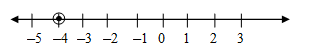On number line, there is only one solution

Infinite solutions

Solution:

The given equation is

2x + 1 = x – 3

2x – x = – 3 – 1

x = –4

Cartesian plane representation: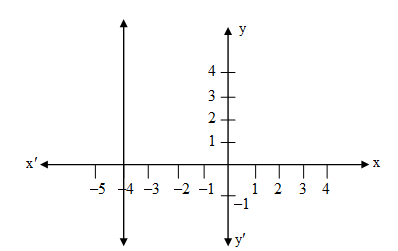There are infinite many points on line x = –4 in Cartesian plane so there are infinite solutions.

## Crack JEE Main with "AI Coach"

• HD Video Lectures
• Unlimited Mock Tests
• Faculty Support#### If the point (3, 4) lies on the graph of 3y = ax + 7, then find the value of a.

Solution:

The given linear equation is 3y = ax + 7 and the point is (3, 4)

If the point (3, 4) lies on the graph of equation 3y = ax + 7 then it will surely satisfy the equation

Put value of x = 3 and y = 4 in equation 3y = ax + 7

3(4) = a(3) + 7

12 = 3a + 7

12 – 7 = 3a

5 = 3a

#### Write the linear equation such that each point on its graph has an ordinate 3 times its abscissa.

It is given that the ordinate is 3 times its abscissa.

That is y = 3x … (i)

Such points are:

 x 0 1 2 y 0 3 6

The points are (0, 0), (1, 3) and (2, 6)

Now plot the graph of equation (1)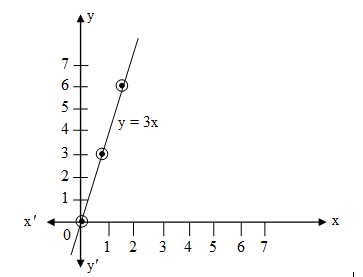Hence y = 3x is the required solution such that each point on its graph has ordinate 3 times its abscissa.

## Crack CUET with india's "Best Teachers"

• HD Video Lectures
• Unlimited Mock Tests
• Faculty Support#### Draw the graph of the linear equation whose solutions are represented by the points having the sum of the coordinates as 10 units.

It is given that sum of the ordinates is 10 units

x + y = 10 …(1)

We can find such points as:

 x 1 2 3 y 9 8 7

The points are (1, 9), (2, 8) , (3, 7) and so on.

Now plotting the graph of the linear equation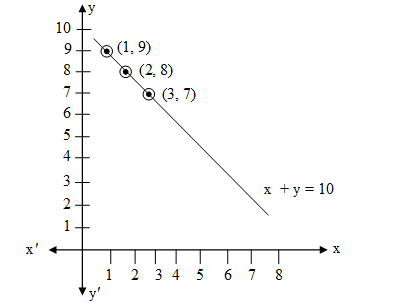#### Draw the graph of the equation represented by a straight line which is parallel to the x-axis and at a distance 3 units below it.

We know that the straight line which is parallel to the x-axis is on the y-intercept, i.e. there is no x-intercept on it.

Now let us plot a straight line on y-intercept at a distance 3 units below the x-axis